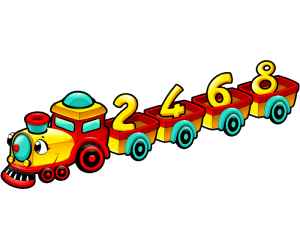Problems
502 - Even Numbers

Even Numbers

Time Limit: 1 sec

The Problem

Even numbers are the numbers which can be divisible by 2. Example: 2, 4, 6, and so on.The Input

The input file contains a series of line. Each line contains a single integer number N (0 <= N <=1001) Input is terminated by EOF.

The Output

For each line of input print the all the even numbers from 0 to N in a separate line.

Sample Input

3

7

Sample Output

0

2

0

2

4

6

Problem Setter: Samia Safa Ahmed